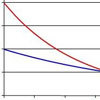#### You may also like### PDF

Given a probability density function find the mean, median and mode of the distribution.### Scale Invariance

By exploring the concept of scale invariance, find the probability that a random piece of real data begins with a 1.### Into the Exponential Distribution

Get into the exponential distribution through an exploration of its pdf.

# Into the Normal Distribution

### Why do this problem?

This problem is based around understanding the probability density function for the normal distribution. The aim is to draw the learner into an understanding of the properties of pdfs without requiring too many complicated calculations: it uses and will reinforce ideas about functions, integration and areas and the use of tables to calculate the probabilities for standardised normal distributions. It will also suit self-motivated independent learners.

### Possible approach

This question could sensibly be used once students are starting to learn about the use of normal distribution tables and standardised normal distributions. There is a lot of scope for numerical estimation of probabilities and the first part could be used to reinforce the fact that a probability density function tells us quite a lot about a distribution even without the need for complicated calculation. It will tie in nicely with other parts of the syllabus on numerical integration.

### Key questions

What do you know about the area under a pdf?
What does the area under a pdf between two points mean?
How might we write down our probabilistic statements in terms of standardised normal variables?

### Possible extension

Can learners find any of the areas enclosed by the lines in the diagrams (using normal distribution tables).
Can they find the points of intersection on the graph?

### Possible support

Encourage learners to rely on their intuitive underestanding of integration in terms of area. Alternatively, focus on the last two parts of the question as a discussion. If they can't come up with their own suggestions of calculation, perhaps they might initially check the estimates of others?